# Angles and measuring them

If you draw two straight lines that cross in some place, you have something like this…X

At the point where the lines cross, there are four gaps, which are called ‘angles‘.

These can be measured – to tell us, for instance, that an angle like this: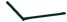is bigger than this: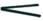.

Obvious, right? Well yes, when the difference is as great as it is in these examples. But if you’re an engineer, or a designer of some sort, very slight differences in measurement can be highly significant.

#### Measuring angles in degrees

It was apparently an Ancient Greek astronomer who decided that angles should be measured in units called ‘degrees’. The same person determined that there should be 360 of them altogether. In other words, if you take a large pie and cut it into 360 equal pieces, each one would have an angle of one degree at the centre. An impossible task, of course. But if you cut the pie into four equal pieces, each one will be a quarter of 360 degrees, which is 90.

What’s more, if you cut the pie into four, you’ll notice that the angle at the centre is like the corner of a square. It has two perpendicular sides, like this: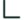This is what’s called a ‘right angle‘. Even if it’s not at the centre of a pie, a right angle always has 90 degrees as its measurement.

#### Measuring angles with a protractor

If you want to measure degrees accurately, the easiest way is to use an inexpensive tool called a protractor. It looks something like this: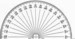.

You can buy them at most stationery shops.

First put the centre point of the protractor on the point of an angle, and the edge along one side. The number around the half circle that matches the position of the other edge of the angle is the number of degrees. Try it first with a square corner, such as a page of a book. You should see that it measures 90 degrees. A smaller angle will have less than 90 degrees and a larger one will have more. It could be any number up to the maximum possible which is 180 degrees – half of 360 –  the ‘angle’ of a straight line.

The concept of angles, and recognising approximate measurements of angles, is a useful one. It may come up naturally in home education. If it doesn’t, you can introduce a protractor casually and show your children how it works. Then suggest they try measuring things. Perhaps they could challenge your division of a cake or pie, and show that one piece really is bigger than another! You could talk with them about how many degrees you would expect each piece to be, depending on how many you divide it into. If you’re dividing into 6, for instance, then you would expect each piece to have a centre angle of 60 degrees.

If your children are intrigued by this idea, you could experiment with checking the angles of various shapes, and seeing if you can spot patterns or principles. For instance, try making various sizes and shapes of triangles, and measuring the angles. A regular triangle has all three angles the same, and all sides the same length. But you can make many types of triangle with different angles and lengths. When you’ve made several, trying adding up the angles of each triangle.

Of course the measurements won’t be exact, but there should be a pattern which emerges. For instance, a regular ‘equilateral’ triangle will have all the angles being 60 degrees. Add those together, and the total is 180. Strangely enough, the same should be true for ANY triangle you make, no matter how irregular. So long as a shape has three straight-edge sides, the total of the angles should make 180. Chances are that if you try this, you’ll get totals like 178 or 184 because it’s difficult to measure exactly, so it’s important to do several in order that your children spot the trend. Alternatively you could try tearing the corners of a triangle, and then placing them like this: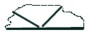to show that they form a straight line – which is 180 degrees.

When you’ve experimented with triangles, you could try shapes with four sides. A square or rectangle has right-angled corners of 90 degrees each, so the total angles will add up to 360 degrees. What about other four-sided shapes such as this: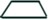or this: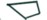?

If you want to know about the regular shapes and what they’re called, see the page on geometric shapes.

For more articles about teaching basic maths at home: